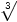.# 分数指数与分数指数幂的运算法则

也叫"根" 或 "有理指数"整数指数，我们首先看整数指数：一个数的指数代表把多少个这个数乘在一起。 例子：82 = 8 × 8 = 64 用文字来表达： 82 可以叫 "8的二次方"、"8的二次幂" 或 "8的平方"

另一个例子：53 = 5 × 5 × 5 = 125

分数指数，如果指数是个分数呢？

 指数是1/2代表平方根 指数是1/3代表立方根 指数是1/4代表四次方根 依此类推！为什么？我们看一个例子来了解为什么，首先，指数定律告诉我们在乘法里怎样处理指数：

我们用分数指数来试试：

### 例子：9½× 9½是什么？

9½ × 9½ = 9(½+½) = 9(1) = 9

√9 × √9 = 9

9½ × 9½ = 9

用另一个分数来试试看，我们再来一次，不过这次指数是（1/4）：

### 例子：16¼

16¼ ×16¼ ×16¼ ×16¼ = 16(¼+¼+¼+¼) = 16(1) = 16

所以，4个 16¼ 相乘的结果是 16，

，故此 16¼ 是 16 的 四次方根

通用规则
从以上我们看到这规则适用于 ½ 和 ¼，其实它是通用的：x1/n = x 的 n次方根

所以规则是：分数指数，像1/n，的意思是 取 n次方根：### 例子：271/3是什么？1/3 =27 = 3

更复杂的分数呢？若分数指数是 43/2，那怎么样？

这实际上是以任何次序做一个 立方（3）和一个 平方根（1/2），听我解释。

整数部分（m），和分数部分（1/n）因为 m/n = m × (1/n)，我们可以这样做：次序并不重要，这样 m/n = (1/n) × m 也可以：我们的结论是：分数指数，像 m/n ,的意思是：   取 m 次幂，然后取 n 次方根 或 取 n 次方根，然后取 m 次幂一些例子：例子：43/2 是什么？

43/2 = 43×(1/2) = √(43) = √(4×4×4) = √(64) = 8

43/2 = 4(1/2)×3 = (√4)3 = (2)3 = 8

答案一样。

### 例子：274/3是什么？?

274/3 = 274×(1/3) =(274) =(531441) = 81

274/3 = 27(1/3)×4 = (27)4 = (3)4 = 81

第二个方法简单很多!

现在来玩玩这个图！
留心看当你在动画里改变分数时，曲线怎样畅顺地变动，显示出分数指数的精髓：

试试这些：

从 m=1 和 n=1 开始，然后慢慢增大 n，留心看 1/2、1/3 和 1/4，接着以 m=2，把 n 上下改变，留心看像 2/3 等等的分数，尝试把指数调成 −1，最后试试把 m 增大，再把 n 减少；然后 减少 m，再 增大 n：曲线会来回绕圈，

.

## 条评论

.

•### 相关文章

••最新评论
•